Spatial Query

2.1具体任务

2.2程序输入

2.3 程序输出

3.1 数据预处理

3.2 Grid-based Spatial Indexing

3.2.1 搜索的特点

3.2.2 范围查询

3.2.3 最邻近查询

3.2.4 基于网格查询的优缺点

3.3 KD-Tree Spatial Indexing

3.3.1 搜索的特点

3.2.2 范围查询

3.2.3最邻近查询

4.1 程序总流程

4.2 具体代码实现

5.1 网格法空间搜索：

5.1.1最邻近算法

5.1.2范围查找算法

5.1.3圆范围查找

5.2 KD-Tree空间搜索

5.2.1最邻近算法查找

5.2.2范围查找

5.2.3半径查找

5.3 索引文件建立时间对比

一、介绍

1.网格索引法：

1. KD-Tree法：

SIFT算法中做特征点匹配的时候就会利用到k-d树。而特征点匹配实际上就是一个通过距离函数在高维矢量之间进行相似性检索的问题。针对如何快速而准确地找到查询点的近邻，现在提出了很多高维空间索引结构和近似查询的算法，k-d树就是其中一种。

三、问题解答

3.1 数据预处理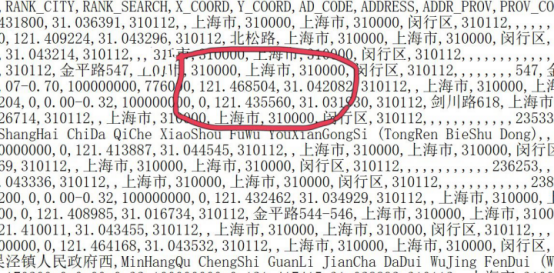图3.1 原始数据文件部分记录示意图

3.2 Grid-based Spatial Indexing

3.2.1 搜索的特点

（1）将空间划分为不相交和均匀的网格

（2）在每个网格和网格点之间建立反向索引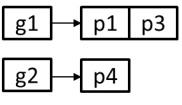3.2.2 范围查询

（1）查找与范围查询相关的网格。

（2）从网格中获取点并确定范围内的点。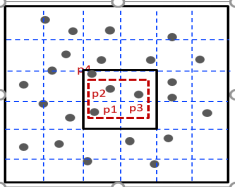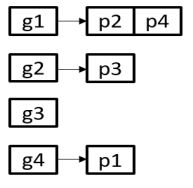3.2.3 最邻近查询

（1）计算的是欧式距离。

（2）道路网络的距离是完全不同的。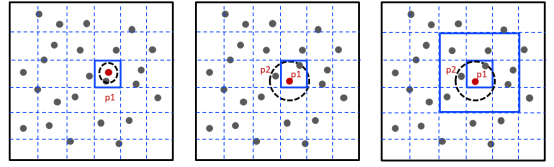最近的点在网格内部          最近的点在网格外部             快速近似

3.2.4 基于网格查询的优缺点

（2）能非常有效的处理范围和最近的查询。

（2）难以处理不平衡的数据。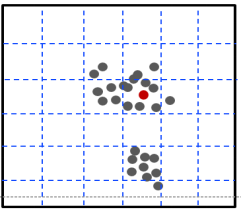3.3 KD-Tree Spatial Indexing

3.3.1 搜索的特点

Kd-Tree，即K-dimensional tree，是一棵二叉树，树中存储的是一些K维数据。在一个K维数据集合上构建一棵Kd-Tree代表了对该K维数据集合构成的K维空间的一个划分，即树中的每个结点就对应了一个K维的超矩形区域（Hyperrectangle）。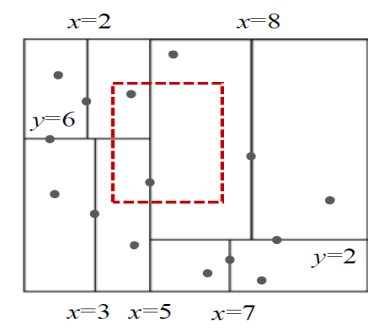3.2.2 范围查询

(1) 判断结点在不在范围之内，如果包含于范围之内就进入子树查询。

(2)通过建立的树节点比较可以节省查询时间，提高查询效率。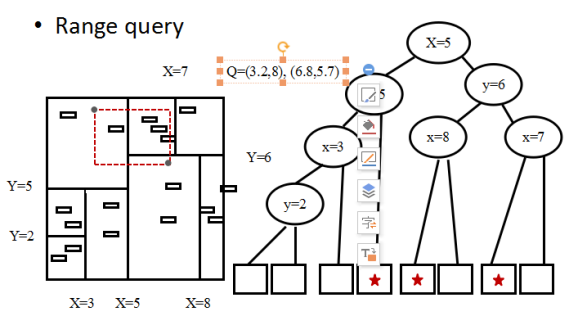3.2.3最邻近查询

(1) 若当前节点比当前最优点更优，则将其作为当前最优节点
(2) 判断左子树是否存在最优点，若有则递归下去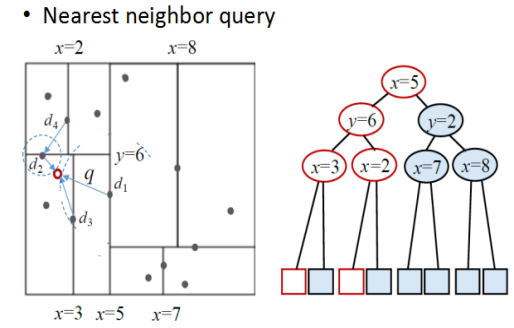四、代码实现

4.1 程序总流程

（1）获取原始点坐标并将其写入到文件中，主要包括读文件和写文件两种操作。

（2）将所选区域等分为格状，读取目标物经纬度，查找网格内部与它最近的点的位置。

（3）若搜寻无果，则扩大搜索范围，采用递归调用的方法逐渐搜寻与他最近的点。

KD-Tree空间搜索：

1.最邻近算法

k_close(p,o,k,)//查询点p,树当前节点o,近邻数目k

(1) 若当前节点比当前最优点更优，则将其作为当前最优节点
(2) 判断左子树是否存在最优点，若有则递归下去

2.范围查找

if v 是叶子

if lc(左子树) 包含于 搜索区域

else 左子树与搜索区域相交

if rc(右子树) 包含于 搜索区域
else 右子树与搜索区域相交

五、程序运行结果

5.1 网格法空间搜索：

5.1.1最邻近算法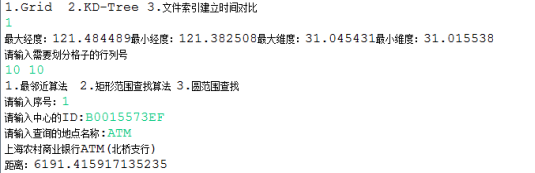5.1.2范围查找算法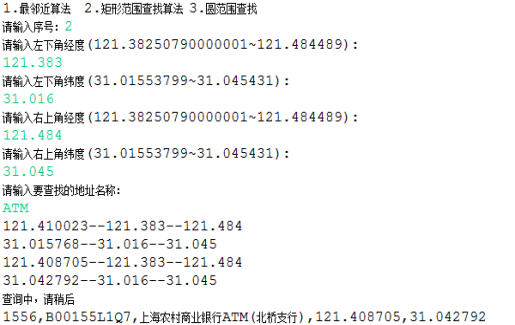5.1.3圆范围查找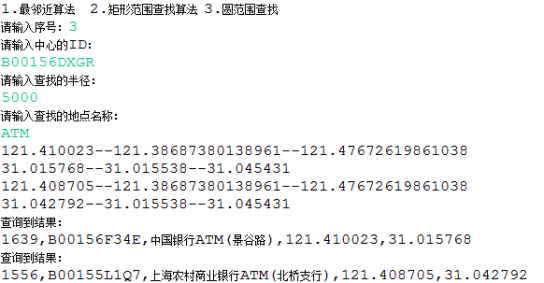5.2 KD-Tree空间搜索

5.2.1最邻近算法查找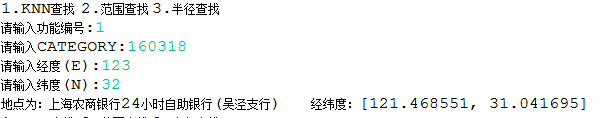5.2.2范围查找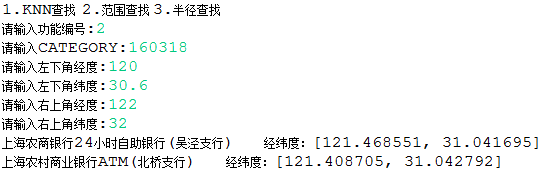5.2.3半径查找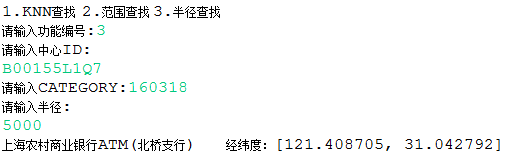5.3 索引文件建立时间对比

抽取的数据放入txt需要频繁读取，所以考虑了两种建立索引的方式，一种是将数据放入对象，然后序列化写入文件，另一种是将数据存入JSON对象，然后写入JSON文件。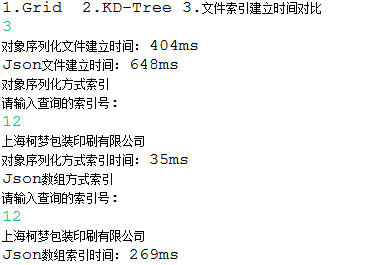public class UtilZ

{

/**

* 读取指定文件指定行号的内容

* @param sourceFile 文件

* @param lineNumber 行号

* @return 字符串内容

*/

public String readAppointedLineNumber(File sourceFile, int lineNumber)

throws IOException {

String s = "";

int lines = 0;

while (s != null) {

lines++;

if((lines - lineNumber) == 0) {

// System.out.println(s);

return s;

}

}

in.close();

return s;

}

/**

* 字符串模糊匹配

* @param text 查询的内容 比如中国银行ATM机

* @return 字符串内容

*/

private static boolean match(String adressName,String text){

Matcher matcher = pattern.matcher(text);

if(matcher.find()){

// System.out.println("匹配到了："+matcher.group(1));

return true;

}

// System.out.println("没有匹配到");

return false;

}

/**

* 根据两点经纬度获得两点距离

* @param lat 经度

* @param lon 纬度

* @return 两点距离

*/

public static double Geodist(double lat1, double lon1, double lat2, double lon2)

{

double delta_lon = Rad(lon2 - lon1);

double top_1 = Math.cos(radLat2) * Math.sin(delta_lon);

double top = Math.sqrt(top_1 * top_1 + top_2 * top_2);

double delta_sigma = Math.atan2(top, bottom);

double distance = delta_sigma * 6378137.0;

return distance;

}

{
return d * Math.PI / 180.0;
}

/**

* 根据某点经纬度和半径，获取圆周内最大最小经纬度

* @param lat 经度

* @param lon 纬度

* @param 半径

* @return 数组minLat, minLng, maxLat, maxLng

*/

public static double[] GetAround(double lat, double lon, int raidus)

{
Double latitude = lat;

Double longitude = lon;

Double degree = (24901 * 1609) / 360.0;

double raidusMile = raidus;

Double dpmLat = 1 / degree;

Double radiusLat = dpmLat * raidusMile;

Double minLat = latitude - radiusLat;

Double maxLat = latitude + radiusLat;

Double mpdLng = degree * Math.cos(latitude * (3.14159265 / 180));

Double dpmLng = 1 / mpdLng;

Double radiusLng = dpmLng * raidusMile;

Double maxLng = longitude - radiusLng;

Double minLng = longitude + radiusLng;

return new double[] { minLat, minLng, maxLat, maxLng };

}

/**

* 求一个一维数组的方差

* @param data 数据

*

* @return 方差

*/

public static double variance(ArrayList<double[]> data,int dimention){

double vsum = 0;

double sum = 0;

for(double[] d:data){

sum+=d[dimention];

vsum+=d[dimention]*d[dimention];

}

int n = data.size();

return vsum/n-Math.pow(sum/n, 2);

}

/**

* 递归实现快速排序算法

* @param data 数据

* @param low 低位置

* @param high 高位置

* @return 方差

*/

public static void QuickSort(double[] data,int low,int high)

{

// TODO 自动生成的方法存根

if(low<high)

{

int middle=GetMiddle(data,low,high);

QuickSort(data,low,middle-1);

QuickSort(data,middle+1,high);

}

}

//将数组拆分

public static int GetMiddle(double[] data, int low, int high)

{

// TODO 自动生成的方法存根

//将数组位置最小的元素赋值给中轴

double temp=data[low];

while(low<high)

{

while(low < high && data[high] >= temp)

{

high--;

}

data[low] = data[high];//比中轴小的记录移到低位置

while(low < high && data[low] <= temp)

{

low++;

}

data[high] = data[low] ; //比中轴大的记录移到高位置

}

data[low] = temp ; //将中轴元素放入中轴

return low;

}

/**

* 求矩形外一点到矩形最小的距离

* @param input 输入的点的数据

* @param max 横纵坐标的最大值

* @param min 横纵坐标的最小值

* @return 最小距离

*/

public static double minP_RDistance(double []input,double []max,double min[])

{

double point_x=input;

double point_y=input;

double max_x=max;

double max_y=max;

double min_x=min;

double min_y=min;

double mindistance=0;

//如果在矩形的左右两边

if(point_y>min_y&&point_y<max_y)

{

if(point_x>max_x)

{

mindistance=Geodist(point_x,point_y, max_x, point_y);

}

else if(point_x<min_x)

{

mindistance=Geodist(point_x,point_y, min_x, point_y);

}

}

else if(point_x>min_x&&point_x<max_x)

{

if(point_y>max_y)

{

mindistance=Geodist(point_x,point_y, point_x, max_y);

}

else if(point_y<min_y)

{

mindistance=Geodist(point_x,point_y, point_x, min_y);

}

}//在四个角上

else

{  //左上

if(point_x<min_x&&point_y>max_y)

{

mindistance=Geodist(point_x,point_y,min_x,max_y);

}//左下

else if(point_x<min_x&&point_y<min_y)

{

mindistance=Geodist(point_x,point_y,min_x,min_y);

}//右上

else if(point_x>max_x&&point_y>max_y)

{

mindistance=Geodist(point_x,point_y,max_x,max_y);

}//右下

else if(point_x>max_x&&point_y<min_y)

{

mindistance=Geodist(point_x,point_y,max_x,min_y);

}

}

return mindistance;

}

}